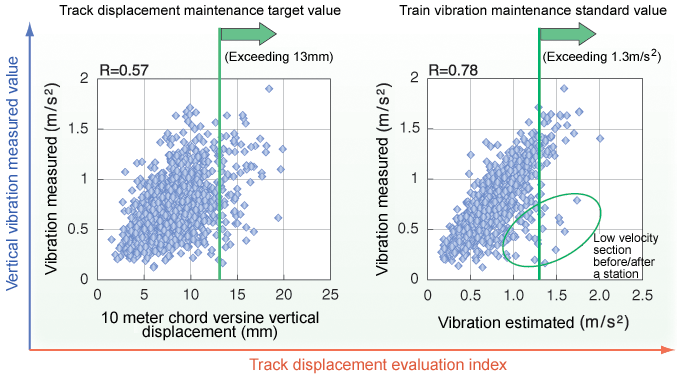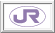3. Evaluation Technique for Track Displacement Based on a Train Vibration-Forecasting Model

In track displacement management, the amount of maintenance necessary is determined by evaluating track displacement using the amplitude of waveforms measured by the track inspection car as an index. However, to improve speed and riding quality on conventional lines, it is more efficient to determine the amount of maintenance by evaluating track displacements using an index with a higher correlation to train vibration. A model was therefore created to estimate vertical and horizontal train vibration using simple calculations from measured waveforms of track displacement, and the model was applied to track displacement evaluation.

Fig.1 shows two graphs for a 120-km track maintenance section on a JR conventional line. The horizontal axes show (a) the 10-meter chord versine vertical displacement waveform (the conventional track displacement evaluation index) and (b) the maximum value for every 100-meter section of the estimated vertical vibration waveform (according to this model), while the vertical axes give the maximum value for every 100-meter section of the measured vertical vibration waveform. The maximum values for each 100-meter section of the estimated vibration waveform shown in Fig.1 (b) correspond closely to the measured values, and clearly show the sections where the train jolts. The number of sections deemed to exceed the standard value for train vibration maintenance will therefore decrease, resulting in reduced maintenance.

The forecasting model will thus allow track condition evaluation corresponding more closely to train vibration than use of the current 10/20 meter chord versine values, and as a result more cost-effective track maintenance can be achieved.(a) 10-meter chord versine vertical displacement (b) Vertical vibration estimated Fig.1 Comparison of the vertical displacement evaluation index and vertical vibration measured values (max. value for every 100-meter section)

HOME > Major Results of Research and Development in Fiscal 2003 > III Comfort/ConvenienceRTRI HOME